### Home > MC2 > Chapter 8 > Lesson 8.2.2 > Problem8-47

8-47.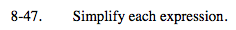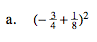Using the order of operations, first simplify the terms in parentheses,
then simplify the terms with exponents.

$\frac{25}{64}$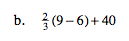Follow the order of operations. First simplify any terms in parentheses, then any exponents, then terms being multiplied or divided, and lastly, combine any terms that are being added or subtracted.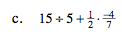See part (b).

$2 \frac{10}{14}$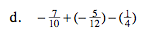Find a common denominator and combine.

$-\frac{164}{120}\text{ or } -1\frac{11}{30}$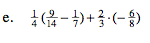See part (b).

$-\frac{3}{8}$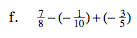See part (d).